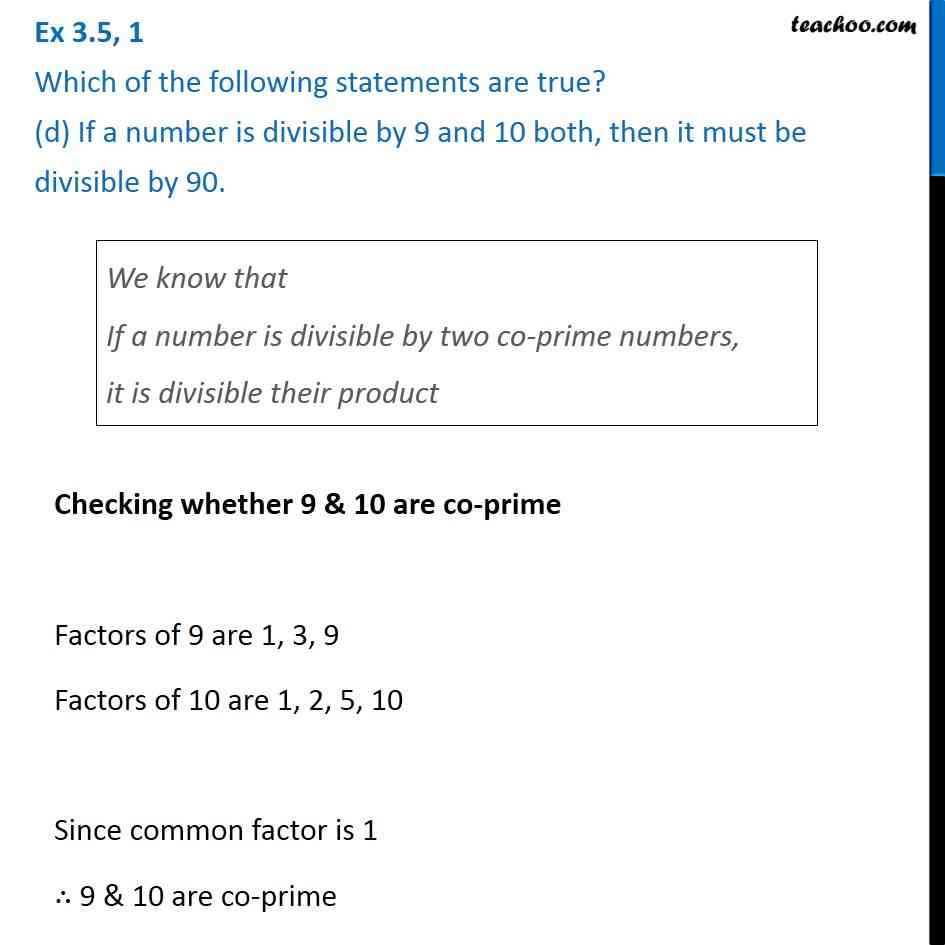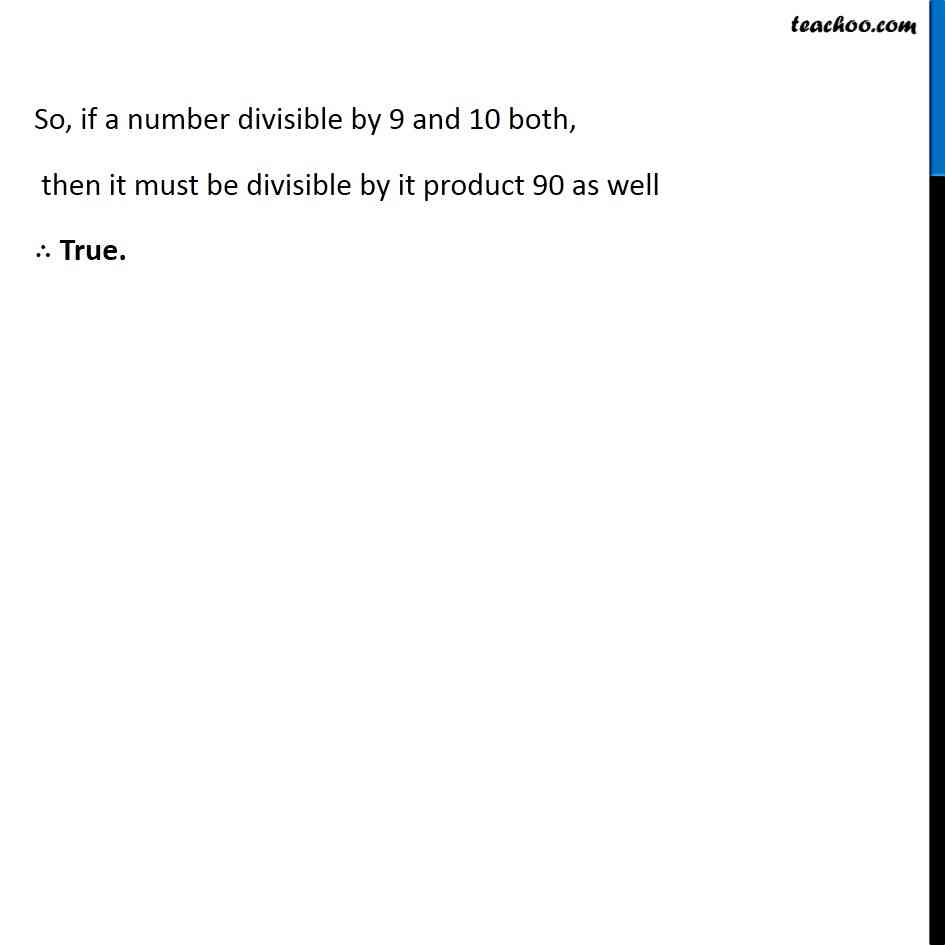Ex 3.5

Chapter 3 Class 6 Playing with Numbers
Serial order wiseLearn in your speed, with individual attention - Teachoo Maths 1-on-1 Class

### Transcript

Ex 3.5, 1 Which of the following statements are true? (d) If a number is divisible by 9 and 10 both, then it must be divisible by 90.We know that If a number is divisible by two co-prime numbers, it is divisible their product Checking whether 9 & 10 are co-prime Factors of 9 are 1, 3, 9 Factors of 10 are 1, 2, 5, 10 Since common factor is 1 ∴ 9 & 10 are co-prime So, if a number divisible by 9 and 10 both, then it must be divisible by it product 90 as well ∴ True.